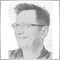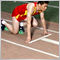new indicator debugging218

hello everybody,

I have some troublein debugging new indicators.

1- the indicator is plotted correctly on historical data but it changes completely when receiving new data. the indicator is simply an exponential moving variance. it seems it starts reading tick by tick instead of using the correct timeframe

2- simlarly during debug the indicator (another one) is plotted correctly, whenever i switch timeframe (ex M1 to H1) the windows colses suddenly without any message, and if i run it outside debugging the plotting is completely different and out fo range.

is there any way to debug at runtime? something like  a print or log of variables value during execution on new data

thanks

p.s. should I attach any code?Documentation on MQL5: Math Functions / MathExp
• www.mql5.com
Math Functions / MathExp - Documentation on MQL517732

michelino:

hello everybody,

is there any way to debug at runtime? something like  a print or log of variables value during execution on new data

You can add Print() statements to print variables wherever you wish,  bear in mind this can fill the log up pretty fast . . .

You can post your code but if you do please use the SRC button to do so . . .  How to use the   SRC   button.2129

An indicator receives every tick, so you have to check for new bars in the indicator. See here for example: https://www.mql5.com/en/articles/37
//---- calculation of starting index first of the main loop
if(prev_calculated==0) // check for the first start of the indicator
first=MAPeriod-1+begin; // start index for all the bars
else first=prev_calculated-1; // start index for the new bars

So it is essential to return the number of consumed bars at the end of the indicator.

return(rates_total);
Regards, UweCustom Indicators in MQL5 for Newbies
• 2010.03.03
• Nikolay Kositsin
• www.mql5.com
Any new subject seems complicated and hard-to-learn for a newbie. Subjects that we know seem very simple and clear to us. But we simply do not remember, that everyone has to study something from scratch, and even our native language. The same is with the MQL5 programming language that offers wide possibilities of developing one's own trading strategies - you can start learning it from basic notions and simplest examples. Interaction of a technical indicator with the MetaTrader 5 client terminal is consider in this article on the example of the simple custom indicator SMA.218

Thanks guys for the quick answers.

I'm going to post the code, it's very short and simple but I cannot understand where is the problem. I'm going to post a screenshot of what's happening

thanks again

Files:39020

michelino:

Thanks guys for the quick answers.

I'm going to post the code, it's very short and simple but I cannot understand where is the problem. I'm going to post a screenshot of what's happening

thanks again

Post your code if you need help. One indicator at a time is better.218

sorry. something went wrong in posting the code. I'm doing it again

//+------------------------------------------------------------------+
//|                                                      EMSIGMA.mq5 |
//|                        Copyright 2013, MetaQuotes Software Corp. |
//|                                              http://www.mql5.com |
//+------------------------------------------------------------------+
#property copyright "Copyright 2013, MetaQuotes Software Corp."
#property version   "1.00"
#property indicator_separate_window
#property indicator_buffers 2
#property indicator_plots   2
//--- plot EMSIGMA
#property indicator_label1  "EMSIGMA"
#property indicator_type1   DRAW_LINE
#property indicator_color1  clrRed
#property indicator_style1  STYLE_SOLID
#property indicator_width1  2
#property indicator_label2  "AVGEMSIGMA"
#property indicator_type2   DRAW_LINE
#property indicator_color2  clrBlueViolet
#property indicator_style2  STYLE_SOLID
#property indicator_width2  1
//--- input parameters
input int      period=20;
input int      spostamento=0;
//--- indicator buffers
double         EMSIGMABuffer[];
double         avgEMSIGMABuffer[]; //long term ema of EMSIGMA
double         emaBuff;
double alpha = 2.0/(period+1.0);
double oalpha = 1.0-alpha;
double emvar = 0;
double ema = 0;
//+------------------------------------------------------------------+
//| Custom indicator initialization function                         |
//+------------------------------------------------------------------+
int OnInit()
{
//--- indicator buffers mapping
SetIndexBuffer(0,EMSIGMABuffer,INDICATOR_DATA);
SetIndexBuffer(1,avgEMSIGMABuffer,INDICATOR_DATA);
//--- sets first bar from what index will be drawn
PlotIndexSetInteger(0,PLOT_DRAW_BEGIN,2*period-2);
//--- sets indicator shift
PlotIndexSetInteger(0,PLOT_SHIFT,spostamento);
//--- name for indicator label
IndicatorSetString(INDICATOR_SHORTNAME,"EMSIGMA("+string(period)+")");
//--- name for index label
PlotIndexSetString(0,PLOT_LABEL,"EMSIMGA("+string(period)+")");
//--- initialization done
//---
return(INIT_SUCCEEDED);
}
//+------------------------------------------------------------------+
//| Custom indicator iteration function                              |
//+------------------------------------------------------------------+
int OnCalculate(const int rates_total,
const int prev_calculated,
const int begin,
const double &price[])
{
//---

if(rates_total<2*period-2)
return(0);
double a;
double aux;
int i;
int start;
if(prev_calculated==0) {
start=period-1+begin;
ema = price[begin];
emvar = 0.0;
EMSIGMABuffer = 0.0;
avgEMSIGMABuffer = 0.0;
emaBuff = emvar;
} else {
start=prev_calculated-1;
}
for(i=start;i<rates_total;i++) {
a = 2.0/(i+1.0);
ema = ema*oalpha+price[i]*alpha;
aux = ema-price[i];
emvar = emvar*oalpha + alpha*aux*aux;
EMSIGMABuffer[i] = MathSqrt(emvar);
emaBuff = emaBuff*(1.0-a) + EMSIGMABuffer[i]*a;
avgEMSIGMABuffer[i] = emaBuff;
}
//--- return value of prev_calculated for next call
return(rates_total);
}
//+------------------------------------------------------------------+39020

michelino:

sorry. something went wrong in posting the code. I'm doing it again

Your indicator use previous value to calculated current value, for example :

emaBuff = emaBuff*(1.0-a) + EMSIGMABuffer[i]*a;

But as ugo58 said you, OnCalculate is called on each tick, so your value are recalculated on each tick. For historical data, on the first call, this is not a problem.218

angevoyageur:

Your indicator use previous value to calculated current value, for example :

But as ugo58 said you, OnCalculate is called on each tick, so your value are recalculated on each tick. For historical data, on the first call, this is not a problem.

so I should use somenthing like this:

iMA(_Symbol,0,1,0,MODE_SMA,_AppliedPrice);39020

michelino:

so I should use somenthing like this:

Why ? That has nothing to do with the problem. The issue isn't about the price but about your calculation algorithm :

for(i=start;i<rates_total;i++) {
a = 2.0/(i+1.0);
ema = ema*oalpha+price[i]*alpha;
aux = ema-price[i];
emvar = emvar*oalpha + alpha*aux*aux;
EMSIGMABuffer[i] = MathSqrt(emvar);
emaBuff = emaBuff*(1.0-a) + EMSIGMABuffer[i]*a;
avgEMSIGMABuffer[i] = emaBuff;
}
This loop is run on EACH TICK, but some of your calculation is expected to be run for EACH BAR. ema, emvar and emabuff variables are all computed based on previous value of the variable. If there are 50 ticks on the last bar, the loop will be run 50 times for the same bar (same value of i). What is your starting value of ema, emvar, emabuff ? The values of the previous tick, but it must be the values of the previous bar.218

angevoyageur:

Why ? That has nothing to do with the problem. The issue isn't about the price but about your calculation algorithm :

This loop is run on EACH TICK, but some of your calculation is expected to be run for EACH BAR. ema, emvar and emabuff variables are all computed based on previous value of the variable. If there are 50 ticks on the last bar, the loop will be run 50 times for the same bar (same value of i). What is your starting value of ema, emvar, emabuff ? The values of the previous tick, but it must be the values of the previous bar.

sorry but now i'm a bit confused...

how can i get the price of the last bar?

why it behaves differently on historicla data?

I understand that onCalculate is run on each tick and not on each bar.

reading the documentation i find this:

int OnCalculate(
const int rates_total,    // number of available bars in history at the current tick
const int prev_calculated,// number of bars, calculated at previous tick
const int begin,          // index of the first bar
const double &price[]     // price array for the calculation
)

and this:

int OnCalculate (const int rates_total,      // size of the price[] array
const int prev_calculated,  // bars handled on a previous call
const int begin,            // where the significant data start from
const double& price[]       // array to calculate
);

is not clear whether price is an array of bars or ticks

reading other indicators some uses iMA ( MACD) some other uses ExponentialMAOnBuffer, since I don't need a full history of EMA I would use just a variable  (double ema, double emvar) to save memory

how would you write these 6 lines to have the indicator working properly?

I'm asking this because an example is often the best way to undertsand how things works

thanks a lot for your helpDocumentation on MQL5: Technical Indicators / iMA
• www.mql5.com
Technical Indicators / iMA - Documentation on MQL539020

michelino:

sorry but now i'm a bit confused...

how can i get the price of the last bar?

why it behaves differently on historicla data?

I understand that onCalculate is run on each tick and not on each bar.

reading the documentation i find this:

and this:

is not clear whether price is an array of bars or ticks

reading other indicators some uses iMA ( MACD) some other uses ExponentialMAOnBuffer, since I don't need a full history of EMA I would use just a variable  (double ema, double emvar) to save memory

how would you write these 6 lines to have the indicator working properly?

I'm asking this because an example is often the best way to undertsand how things works

thanks a lot for your help

The price is not a problem, the array provided with OnCalculate gives you price for each bar.

As I explained in my previous, and I can't be more clear than that, you have rewrite your calculations. Do you understood what I explained in this post ? You don't have to save all ema values, but at least this of the last bar.